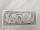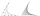# Equation

$-3\left(2-x\right)-x=2\left(3x+1\right)+2x$

x =  -1.3333

### Step-by-step explanation:

-3(2-x)-x=2(3x+1)+2x

-3•(2-x)-x=2•(3•x+1)+2•x

6x = -8

x = -4/3 ≈ -1.333333

Our simple equation calculator calculates it.Did you find an error or inaccuracy? Feel free to write us. Thank you!Tips to related online calculators
Do you have a linear equation or system of equations and looking for its solution? Or do you have a quadratic equation?

## Related math problems and questions:

• My fatherMy father has a big farm. 6/8 of it were planted with mango trees, 1/2 of the remainder are guava trees and the rest 10 trees are santol trees. What is the number of all trees?
• Profit, revenue, costProfit, P(x), is the difference between revenue, R(x), and cost, C(x), so P(x) = R(x) - C(x). Which expression represents P(x), if R(x) = 3x3 + 2x - 1 and C(x) = x4 - x2 + 2x + 3?
• Equation 29Solve next equation: 2 ( 2x + 3 ) = 8 ( 1 - x) -5 ( x -2 )
• EquationFind x: x + 1/2 = 1/3
• EquationsSolve following system of equations: 6(x+7)+4(y-5)=12 2(x+y)-3(-2x+4y)=-44
• Eq2 equationsFor each of the following problems, determine the roots of the equation. Given the roots, sketch the graph and explain how your sketch matches the roots given and the form of the equation: g(x)=36x2-12x+5 h(x)=x2-4x+20 f(x)=4x2-24x+45 p(x)=9x2-36x+40
• Evaluate expr with 2 varsIf a=6 and x=2, find the value of (2ax + 7x- 10) /(4ax-3a-2)
• Simply equationSolve this equation for x: 9x-6(x-1)=5(x+2)-11
• Equation 25Solve following simple equation: 3/4(x+5)=1/2(x+9)
• Polynomial rootsFind the other zeroes of the polynomial 2x4+3x3-3x2-6x-2, if two of them are root2 & -root2
• Non linear eqsSolve the system of non-linear equations: 3x2-3x-y=-2 -6x2-x-y=-7
• Simple equationSolve the following simple equation: 2. (4x + 3) = 2-5. (1-x)
• MartinaMartina is solving the equation 4x - 11 = 2x + 391. Here are the first steps of her solution. 4x - 11 = 2x + 391 2x - 11 = 391 2x = 402 What did Martina do to get 2x - 11 = 391?
• Simple equation 1035= 7*3*x what is x?
• Find xSolve: if 2(x-1)=14, then x= (solve an equation with one unknown)
• EquationSolve equation and check the result: 1.4x - 3/2 + x - 9,8 = x + 0,4/3 - 7 + 1,6/6
• Consider 2Consider the following formula: y = 3 ( x + 5 ) ( x - 2 ) Which of the following formulas is equivalent to this one? A. Y=3x2+9x-30 B. Y=x2+3x-10 C. Y=3x2+3x-10 D. Y=3x2+3x-30